# Class 10 Light Reflection and Refraction Notes

Light Reflection and Refraction Notes

## Reflection of Light:-

The phenomenon of bouncing back of a ray of light after striking on a highly polished surface is called reflection of light.

## Laws of Reflection:-

• First law:- The angle of incidence is equal to the angle of reflection. i.e., ∠i = ∠r
• Second Law:- The incident ray, the normal at the point of incidence and the reflected ray, all lie in the same plane.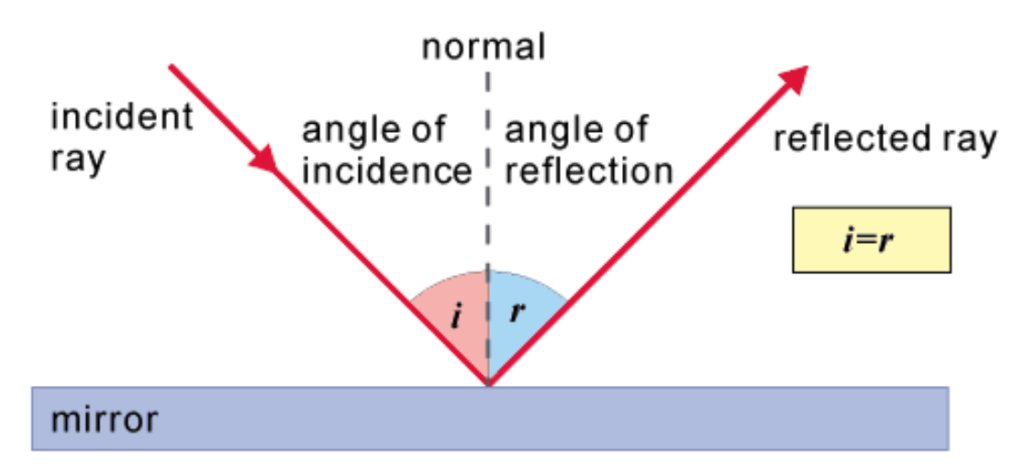## Properties of image formed by a plane mirror:-

• virtual
• erect
• same size as the object
• formed at the same distance as the object from the mirror
• laterally inverted

➢Focal length of plane mirror is infinity.

## Spherical Mirror:-

The mirror whose reflecting surface is a part of sphere is called spherical mirror.

#### Concave Mirror (Converging Mirror):-

The spherical mirror whose reflecting surface is curved inwards is called concave mirror.

#### Convex Mirror (Diverging Mirror):-

The spherical mirror whose reflecting surface is curved outwards is called convex mirror.

Pole(P):- The centre of the reflecting surface of spherical mirror is called pole.

Centre of Curvature(C):- The centre of the sphere of which the spherical mirror is a part is called the centre of curvature.

Principal Axis:- An imaginary line passing through the centre of curvature and pole is known as principal axis.

Radius of Curvature(R):- The radius of the sphere of which the spherical mirror forms a part, is called radius of curvature. OR, The distance between pole and centre of curvature is called radius of curvature.

Principal Focus(F):- The point on the principal axis at which the reflected rays meet or appear to meet when the incident rays are parallel to principal axis is known as principal focus of the mirror.

Focal Length(f):- The distance between the pole and the principal focus of a spherical mirror is called the focal length.

Relation between ‘R’ and ‘f’:- R = 2f

Aperture:- The diameter of the reflecting surface of spherical mirror is called its aperture.

## Ray diagrams of rays used to form the image in spherical mirrors:-

Rule 1:-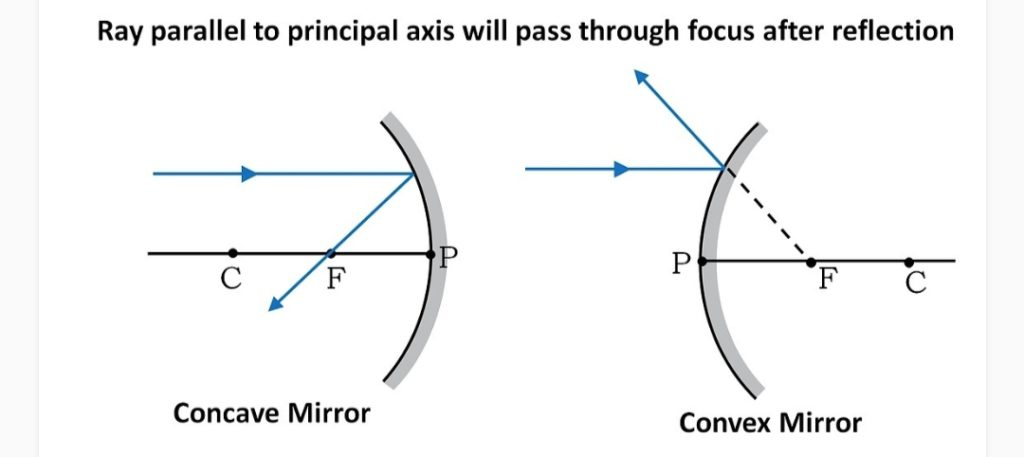Rule 2:-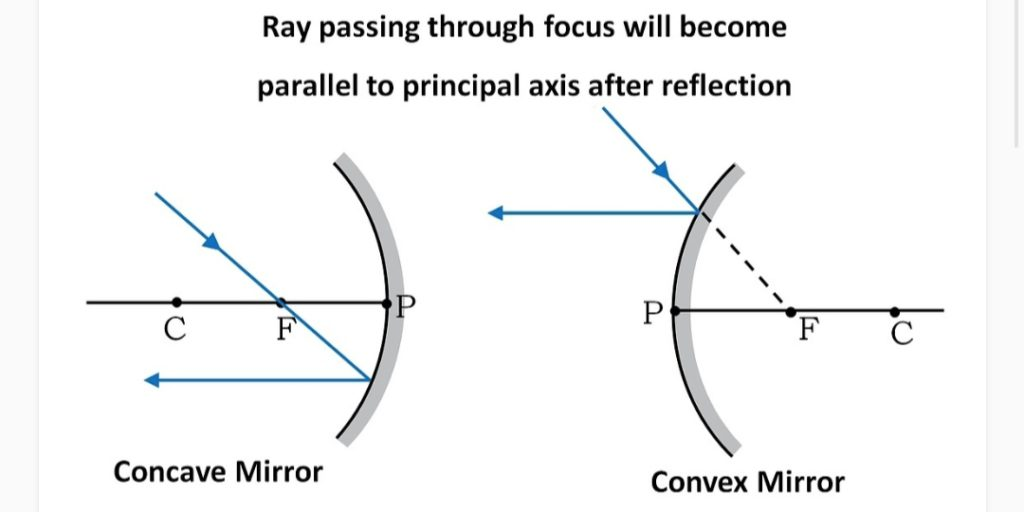Rule 3:-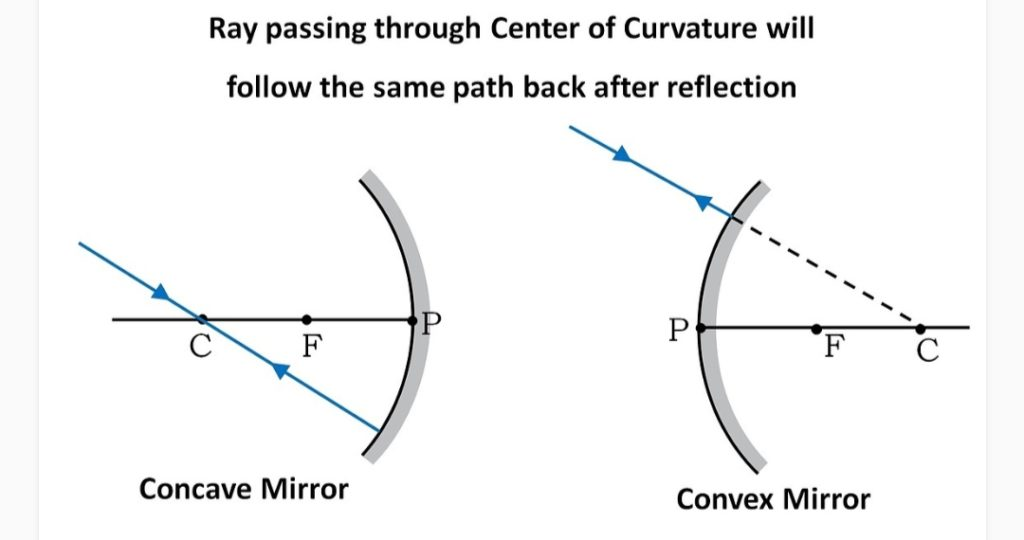Rule 4:-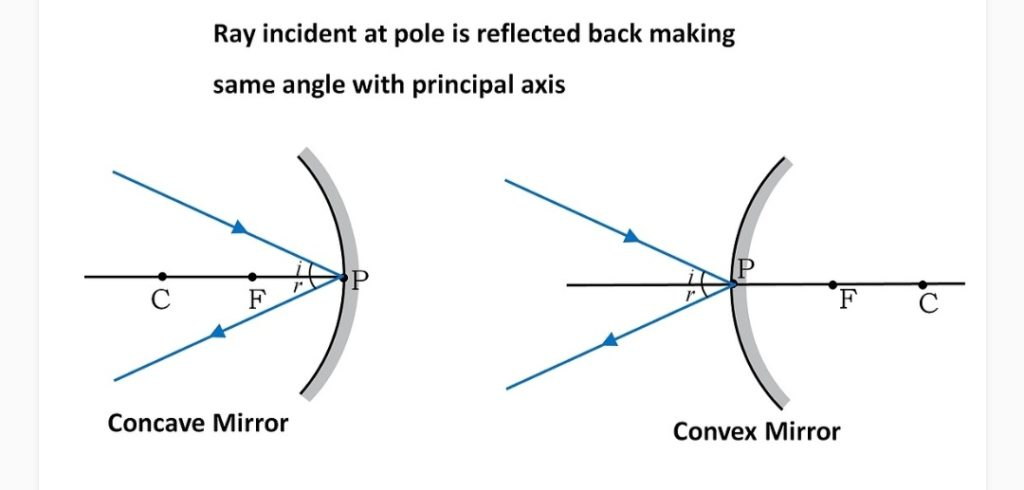## Uses of Concave Mirror:-

• It is used in torches, search-lights, vehicles headlights to get powerful beams of light.
• It is used as shaving mirror.
• It is used by dentists to see large image of teeth.
• It is used in solar furnaces to concentrate sunlight to produce heat.

## Uses of Convex Mirror:-

• It is used as rear view mirror in vehicles.
• It is used by shopkeepers to see the complete image of shop.

Mirror Formula:- 1/f = 1/v + 1/u

Magnification:- m = -v/u Or, m = hi/ho

## Sign Convention for Reflection by Spherical Mirrors:-

• If ‘m’ is negative then, image is real and inverted.
• If ‘m’ is positive then, image is virtual and erect
• If |m|>1 then, image size is magnified.
• If |m|=1 then, image size is equal to image size.
• If |m|<1 then, image size is diminished.

## Refraction of Light:-

The phenomenon of bending of a ray of light when it passes from one medium to another medium is called refraction of light .

• In going from rarer to denser medium, the ray of light bends towards normal and in going from denser to rarer medium, the ray of light bends away from normal.
• No refraction occurs, when (i) light is incident normally on a boundary, (ii) refractive indices of the two media in contact are equal.

## Refraction of light through a glass slab:-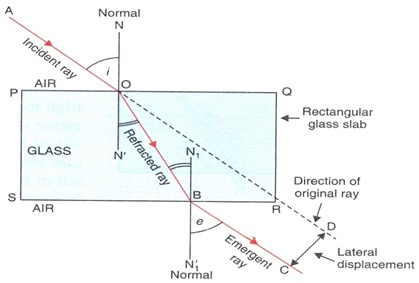Lateral Displacement:- The perpendicular distance between emergent ray and incident ray produced forward is called lateral displacement.

## Laws of refraction of light :-

• First law :- The incident ray, the refracted ray & the normal at the point of incidence, all lie in the same plane.
• 2nd law(Snell’s law):-The ratio of sine of angle of incidence to the sine of angle of refraction is constant for a given color of light & for a given pair of media.

i.e. sin i/sin r = constant

## Refractive Index:-

• Relative refractive index of medium 2 w.r.t. medium 1 (n21) = Sin i/Sin r
• n21 = Speed of light in medium 1/Speed of light in medium 2 = v1/v2
• n12 = v2/v1
• n12 = 1/n21 Or, n12 x n21 = 1
• Absolute refractive index of a medium = Speed of light in air/Speed of light in the medium Or, nm = c/vm
• Speed of light in air (c) = 3 x 108 m/s
• n21 = n2/n1 Or, n12 = n1/n2
• n31 = n32 x n21

## Lens:-

A lens is a transparent medium bounded by two surfaces, of which one or both surfaces are spherical.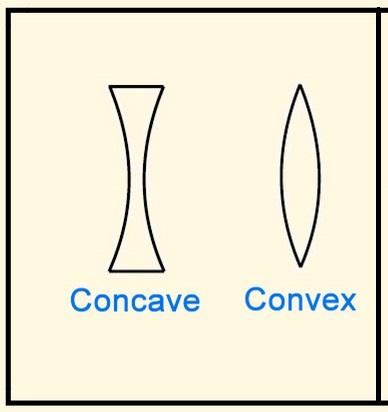Centres of Curvature:- The centres of spheres of which the two spherical surfaces of the lens are the parts are called its centres of curvature.

Principal Axis:- An imaginary straight line passing through the two centres of curvature of a lens is called its principal axis.

Optical Centre(O):- The central point of a lens is called its optical centre.

Aperture:- The effective diameter of the circular outline of a spherical lens is called its aperture.

Principal Focus:- The point on the principal axis at which refracted rays meet or appear to meet when the incident rays are parallel to the principal axis is called principal focus.

## Ray Diagrams of Rays Used to Form the Image in Spherical Lenses:-

Rule 1 :-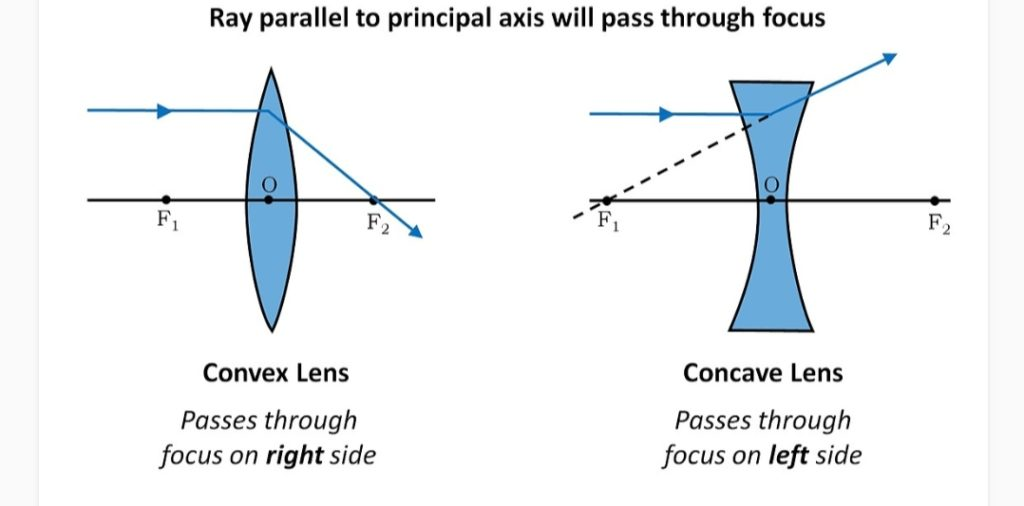Rule 2:-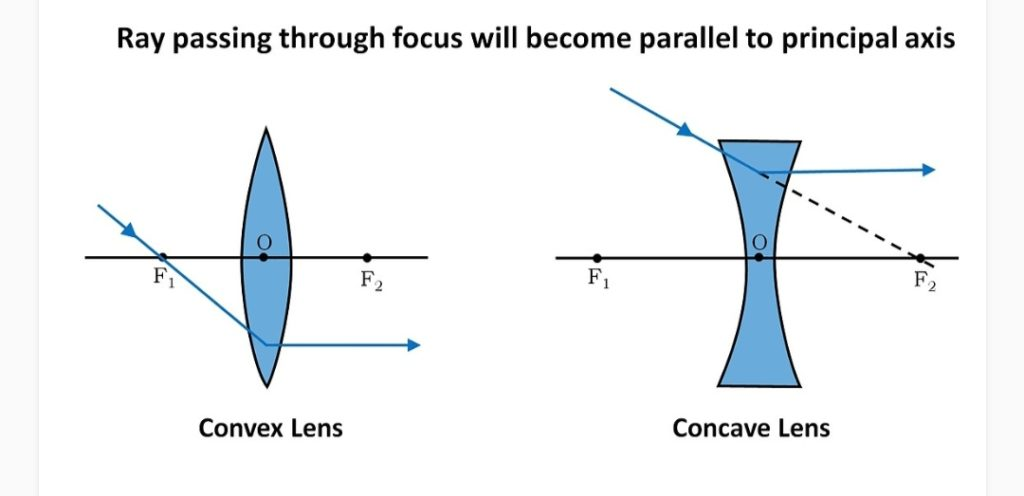Rule 3:-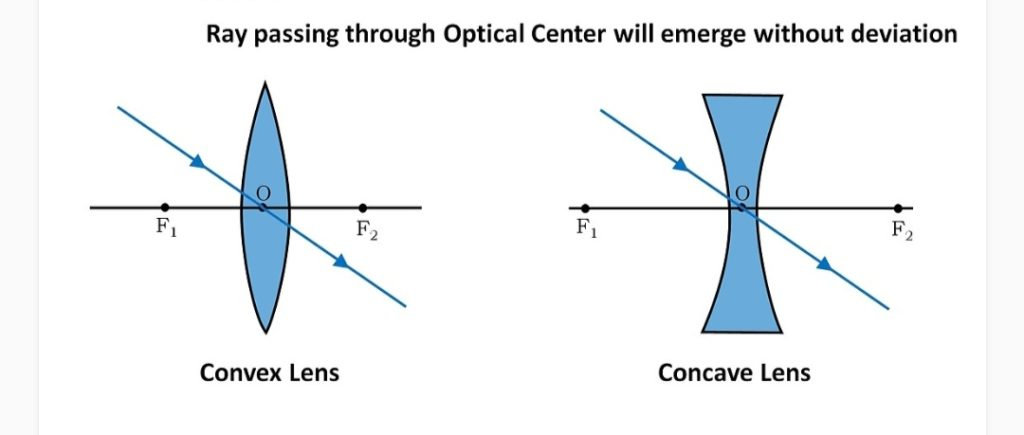## Ray Diagrams of Image Formation by Concave Lens:-

Lens Formula:- 1/f = 1/v- 1/u

Magnification:- m = v/u Or, m = hi/ho

## Power of a lens:-

The degree of convergence or divergence of light rays by a lens is called its power. Or, The reciprocal of focal length (in meter) of a lens is called its power.

• P = 1/f(in m)
• S.I. unit:- Dioptre (D)
• Power of the combination of lenses is: P = P1 + P2 + P3 +……+ Pn